# 1.思维导图及学习体会

## 1.1思维导图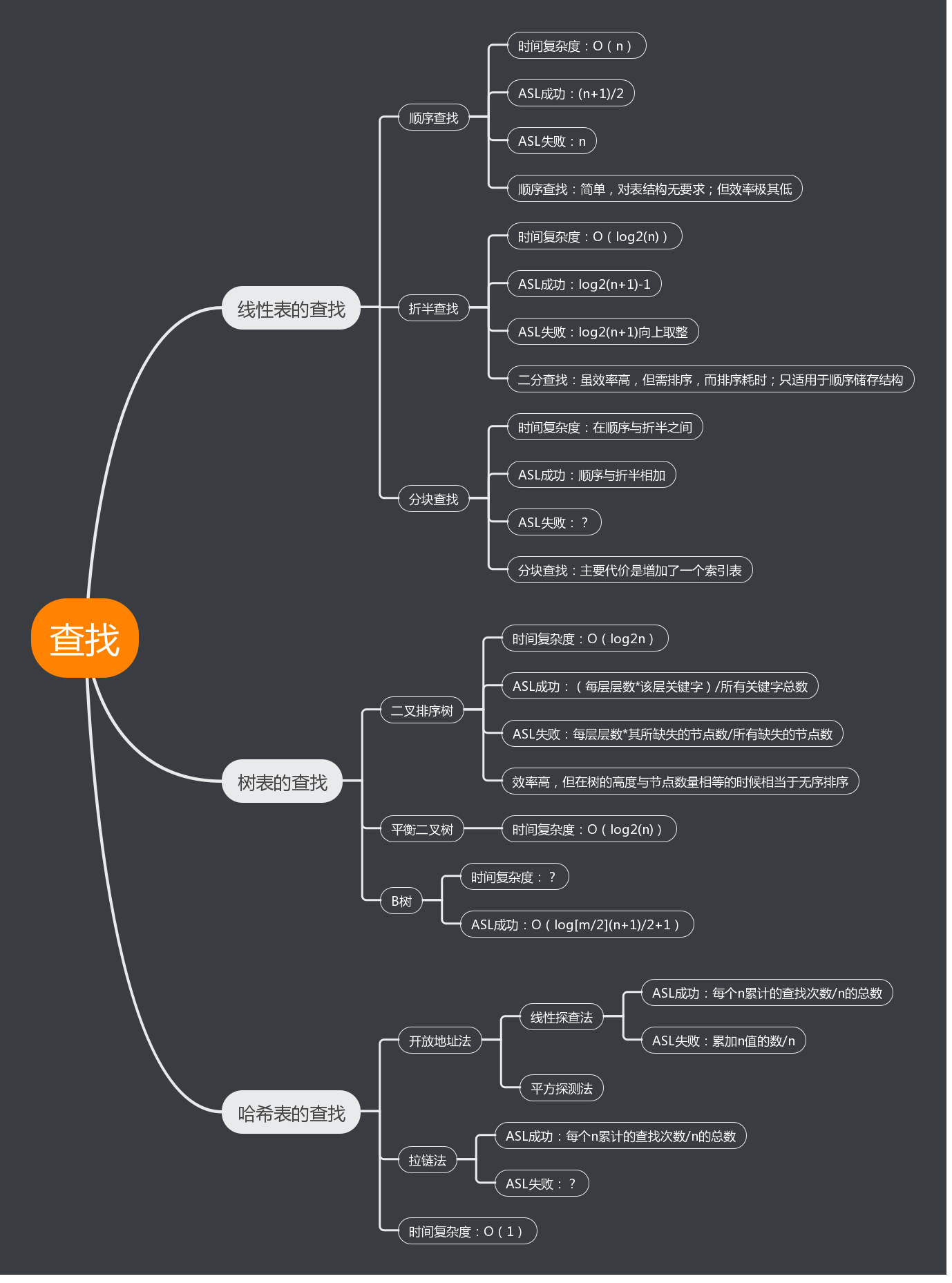## 1.2谈谈你对查找运算的认识及学习体会

1.个人认为本章的重点在于分辨各类查找的时间复杂度与ASL，是非常依赖前面学习的一章。对于我个人而言各类查找的分门别类记忆是有难度的，我并不能较好的将其区分开，特别是哈希表的相关知识。
2.线性表的查找相对来说简单多了，主要是它存储结构是线性的、逻辑比较容易理解，操作起来也比较容易。而且线性表有点类似数组那样，所以我个人觉得线性表的查找会简单点。
3.学到树表的查找后，内容就现相对有点多，而且也不太容易理解，二叉搜索树、平衡二叉树、AVL树、B-树、B+树，各种查找树，不仅逻辑上复杂，操作及代码的编写也复杂，还有各种ASL的计算，平衡二叉树的插入删除调整等等，所以学起来挺困难的，花了挺多时间。比较长的时间是去理解。
4.再到哈希表的查找，内容是相对较少，但是却不好理解，单单是建表就不简单，要花很多的时间去理解，还有解决哈希冲突的方法、ASL的计算等等，这些是最难受的，每学到一个新的知识，就要去了解它的代码，再加上上课的速度快，所以最近有点赶不上。
5.总之，树表与哈希表的查找相比线性表难度大了很多，需要花更多的时间去学习。只有了解了它的本质，那么代码才写得出来。


# 2.PTA实验作业

## 2.1.题目1：6-3 二叉搜索树中的最近公共祖先

### 2.1.1设计思路（伪代码）

int find(Tree T, int x)
{
if   T为空   then   return 0
end if
if   x 等于关键字  then
return 1
else if    x   大于  关键字
调用函数find(T->Right, x)
else
调用函数find(T->Left, x)
end if
}
int LCA(Tree T, int u, int v)
{
if   T  为空  then   返回ERROR
end if
if   find(T, u) 返回0或者find(T, v)返回0   then     返回ERROR
end if
if   T->Key 大于等于u并且T->Key小于v或者 T->Key 小于等于 u并且T->Key 大于等于 v   then
返回 T->Key
if   u > T->Key  then
LCA(T->Right, u, v)
else if   u < T->Key   then
LCA(T->Left, u, v)
}


### 2.1.2代码截图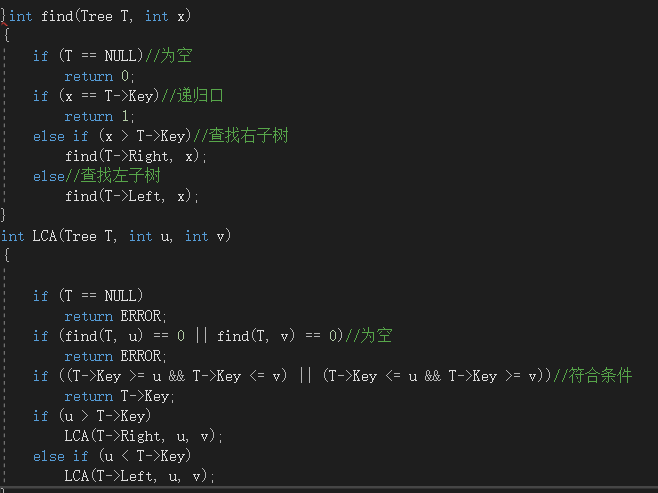### 2.1.3本题PTA提交列表说明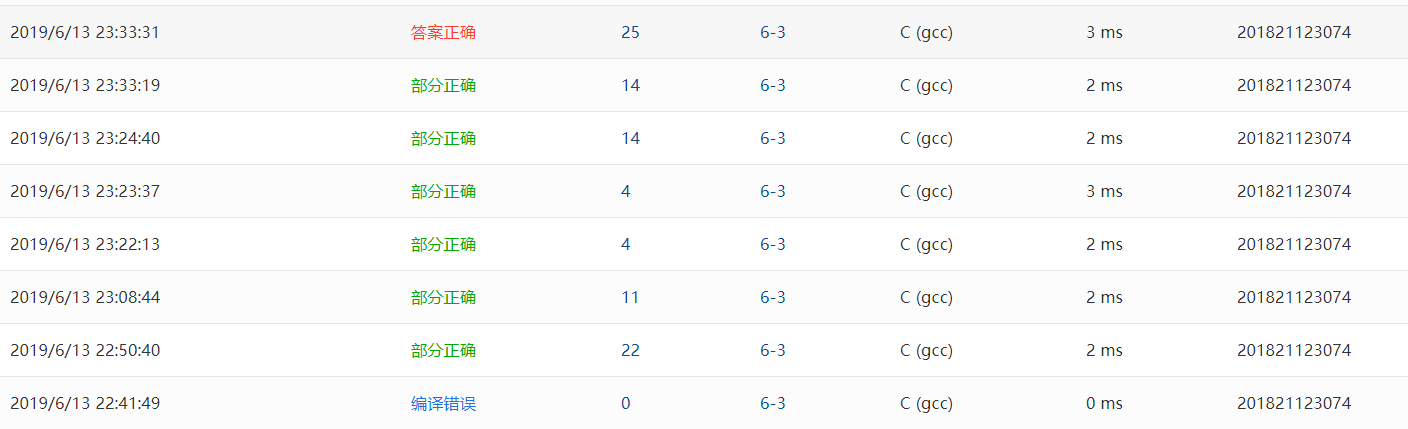• Q1:刚开始提交有出现错误，测试点提示不在树中的情况错误，原来是忽略了不在树中情况，后面为了还是用递归的方法。
• A1:添加了一个判断节点是否在树中的find函数，修改完后提交正确。
• Q2:老师在课上有讲解如何写，就是利用到了二叉搜索树的性质来解决该问题。
• A2:左子树的结点的值都小于根结点的值，右子树的结点的值都大于根结点的值，判断给出的俩个值是不是一个大于一个小于，如果是则这时的根结点就符合要求。

## 2.2.题目2：6-1 二叉搜索树的操作集

### 2.2.1设计思路（伪代码）

Position FindMin(BinTree BST)
{
if  BST  不为空  then
if   BST的左孩子不空   then
递归调用函数 FindMin(BST->Left)
end if
else
返回BST
end if

}
Position FindMax(BinTree BST)
{
if   BST 不为空   then
if   BST右孩子不为空   then
while  BST右孩子不为空
BST = BST->Right
end while
end if
end if
返回 BST
}

BinTree Insert(BinTree BST, ElementType X)
{
if   BST为空  then
BinTree p
p = (BinTree)malloc(sizeof(struct TNode))
X存入p的数据域
p的左右孩子都置为空
BST = p
else if   X小于  BST->Data
BST->Left = Insert(BST->Left, X)
else if   X大于  BST->Data
BST->Right = Insert(BST->Right, X)
end if
返回 BST
}
BinTree Delete(BinTree BST, ElementType X)
{
if   BST为空  then
else
if   X小于 BST->Data   then
BST->Left = Delete(BST->Left, X)
else if   X大于  BST->Data
BST->Right = Delete(BST->Right, X)
else if   X等于BST->Data
if   BST的左右孩子都不为空   then
BinTree p
p = FindMin(BST->Right）
BST->Data = p->Data
BST->Right = Delete(BST->Right, BST->Data)
else
if   BST的左孩子为空   then   BST = BST->Right
else if   BST右孩子为空   BST = BST->Left
end if
end if
end if
返回 BST
}

Position Find(BinTree BST, ElementType X)
{
if   BST == NULL   then
return NULL
if   BST->Data == X   then
return BST
else if   X < BST->Data   then
return Find(BST->Left, X)
else if   X > BST->Data   then
return Find(BST->Right, X)
return BST
}


### 2.2.2代码截图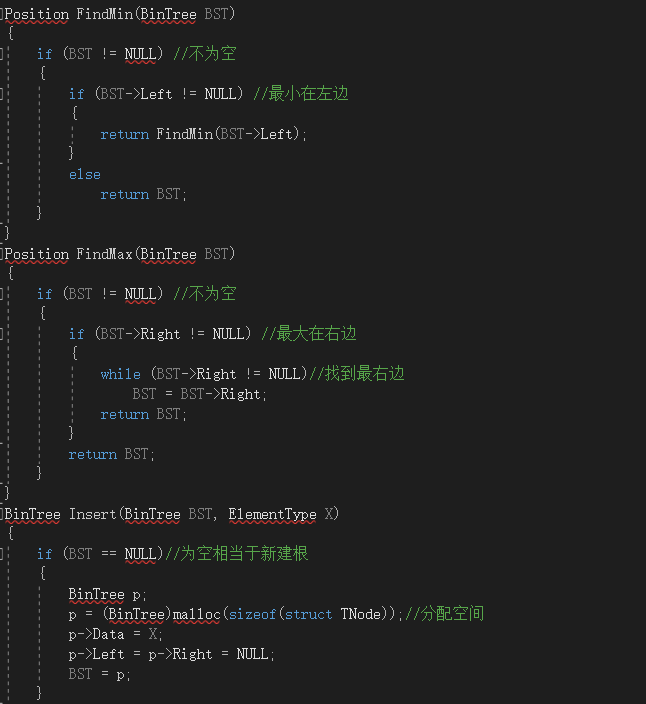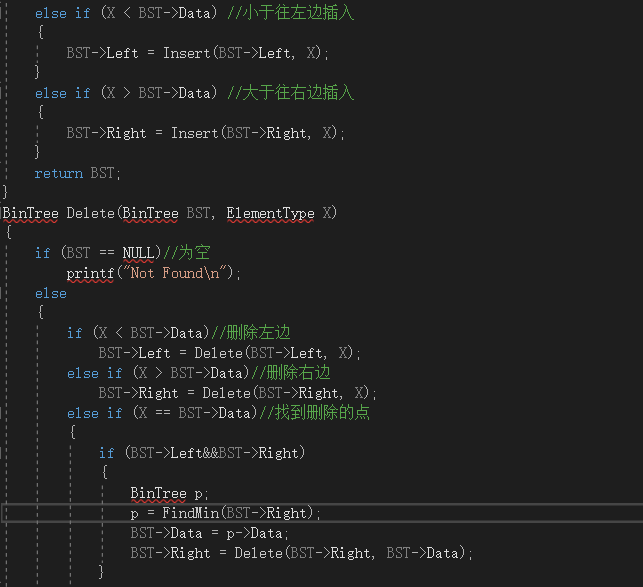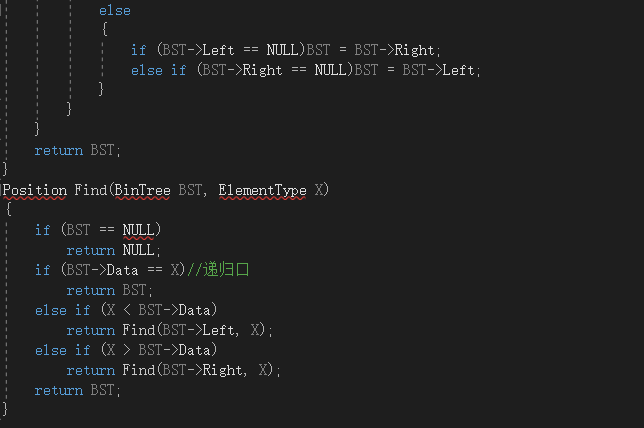### 2.2.3本题PTA提交列表说明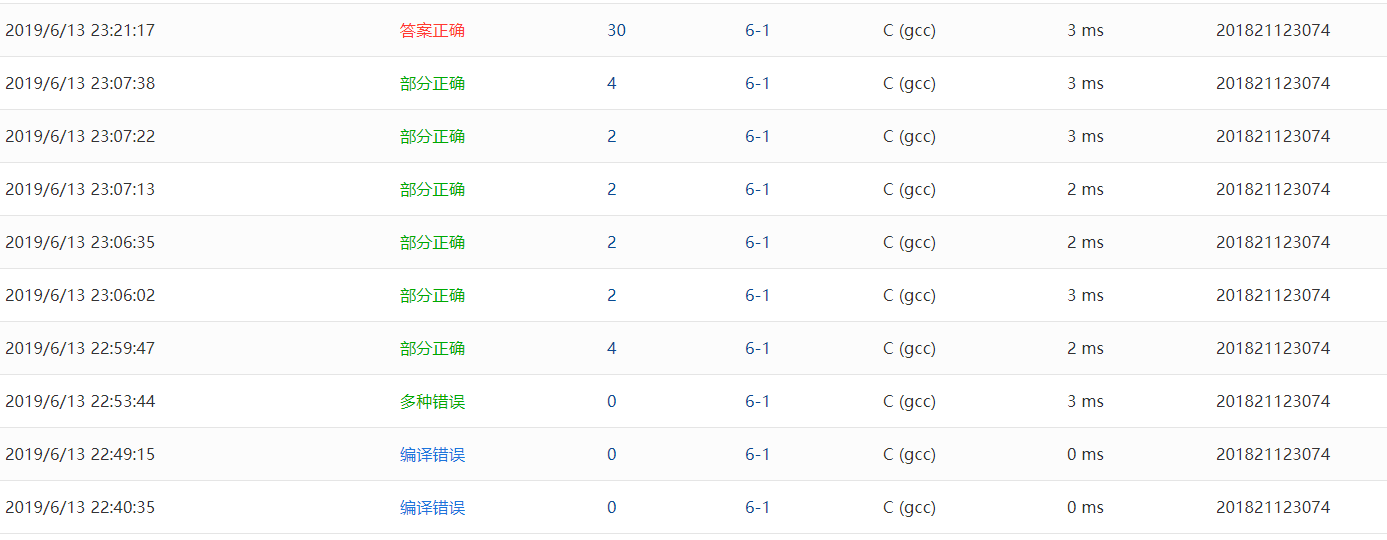• Q1:一开始提交有出现查找超时的情况。通过用测试数据调试查找不到的元素。
• A1:调了好久后发现是在给所有哈希中count赋值零时，将哈希长度弄错为n，改为m就对了。
• Q2:提交的时候一直答案错误，找了好久看不出来，就找大佬来看看，马上就看出来了，因为我们是一样的错误。
• A2:理解错误查找失败的查找次数，全局变量定义的uns_count是用来计算查找不成功次数，添加到不成功的判断条件下就可以了。

## 2.3.题目3：7-1 QQ帐户的申请与登陆

### 2.3.1设计思路（伪代码）

#include <bits/stdc++.h>
#include<iostream>
#include<map>
#include<string>
int main()
定义整数n,m
定义字符串 a,b,c
set<string> match
map<string,string> iter
输入n
while   n大于0且n自减  do
输入 a、b、c
if   a等于L   then
if   match.find(b)等于match.end()   then
输出ERROR: Not Exist
else
if   iter[b]不等于c   then
输出ERROR: Wrong PW
else
end if
else if   a等于N
if   match.find(b)不等于match.end()   then
输出ERROR: Exist
else
输出New: OK
iter[b]=c
match.insert(b)
end if
end if
end while


### 2.3.2代码截图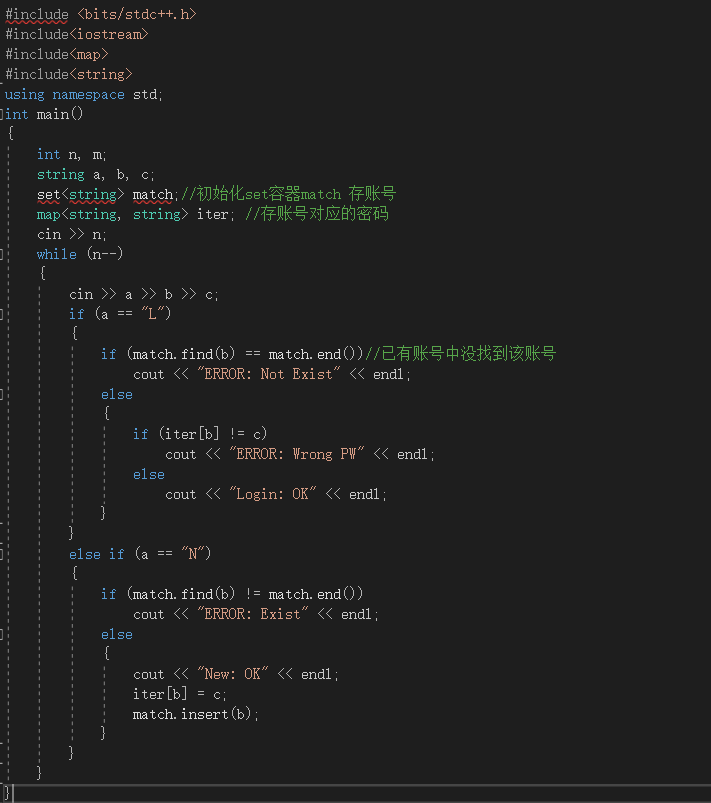### 2.3.3本题PTA提交列表说明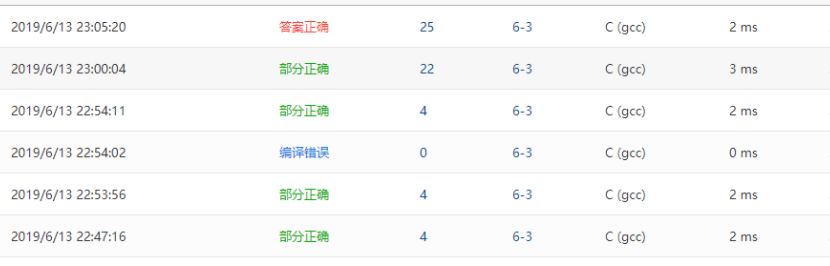• Q1:一开始写的时候定义了个数组用来存放QQ号和密码的，但是提交上去发现有个测试点事有关于上下界的问题。
• A1:我就想是不是因为设置的时候过小了，所以修改了数组的大小再交上去过了那个测试点，还有字符数组末尾为\0也是要记住的。
• Q2:后面我写出来，但是却一直错误，虽然答案输出来是对的，找了大佬来帮忙看一下，也没看出来，我就借鉴他们的写法。
• A2:原来他们用了map容器，所以代码量较少许多，也有许多步骤简单了许多。

# 3阅读代码

## 3.2 解题思路

• 首先判断键key是否为null,若为null,则调用putForNullKey(V v)方法完成put，如果key不为null了，先调用hash(int)方法，计算key.hashCode的hash值，再调用indexFor(int,int)方法求出此hash值对应在table数组的哪个下标i上 (bucket桶)，遍历bucket桶上面的链表元素，找出HashMap中是否有相同的key存在，若存在，则替换其value值，返回原来的value值，若元素e.hash值和上面计算的hash值相等，并且(e.key == 入参key,或者入参key equals 相等 e.key)，则说明HashMap中存在了和入参相同的key了，执行替换操作；在执行替换操作的时候，调用Entry对象的recordAccess(HashMap<K,V> m)方法，程序走到这，说明原来HashMap中不存在key,则直接将键值对插入即可，由于插入元素，修改了HashMap的结构，因此将modeCount+1，调用addEntry(int,K,V,int)方法进行键值对的插入，由于原来HashMap中不存在key，则不存在替换value值问题，因此返回null。

## 3.3 代码截图

public V put(K key, V value) {

if (key == null)
return putForNullKey(value);

int hash = hash(key.hashCode());

int i = indexFor(hash, table.length);

for (Entry<K,V> e = table[i]; e != null; e = e.next) {
Object k;

if (e.hash == hash && ((k = e.key) == key || key.equals(k))) {
V oldValue = e.value;
e.value = value;

e.recordAccess(this);
return oldValue;
}
}

modCount++;